# Measurement error models

Part I: Estimation in regression models with errors in covariates

# 1Measurement error models

Consider an ordinary model of nonlinear regression

Here, ξiRd are known (observable) values of regressors;

β is an unknown vector of regression parameters that belongs to the parameter set ΘRp;

f : Rd × ΘR is a known (given) regression function;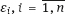are random observation errors usually assumed to be independent, (i.e., with zero mean), and having finite variance;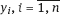are observable values of the dependent variable, or response.

The regression parameter β should be estimated in frames of the model (1.1) by observations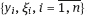.

As another example of regression model, consider a binary logistic model. Let the response yi take two values, 0 and 1, depending on the true value of the regressor ξi, namely

where

is the odds function and β = (β0, β1)T is the regression parameter. The observed couples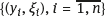are assumed stochastically independent, and the parameter β should be estimated by the observations.

The model (1.2) is widely used in epidemiology and can be interpreted as follows. yi is an indicator of disease for the subject i of a cohort; in the case yi = 1, the subject i has obtained a given type of disease during a fixed period of observations; in the case yi = 0, the subject i has not demonstrated any symptoms of the disease during the period; ξi is the true value of the regressor that affects on disease incidence.

Another model of the odds function is widely used in radio-epidemiology, namely, linear one

The model (1.2) and (1.4) serves for the simulations of the incidence rate of oncological diseases caused by radiation exposure; the regressor ξi is the radiation dose recieved by the subject i of the cohort during the fixed period of observations. This period includes also all the registered cases of cancer.

The parameters β0 and β1 to be estimated are the ones of radiation risk. From mathematical point of view, the model (1.2) and (1.4) is a generalized linear model (GLM) of binary observations; it resembles a logistic model (1.2) and (1.3) but somewhat differs from it. Herein the term “generalized linear model” means that the parameters of the conditional distribution yi|ξi depend on the regressor ξi through a linear expression β0 + β1ξi, where β0 and β1 are unknown regression parameters. Of course, the logistic model (1.2) and (1.3) is also a generalized linear regression model.

The main object of this book is a model with errors in the regressor. It is called also errors-in-variables model or measurement error model.

A distinguishing feature of such models is that the true value of the regressor ξi is unknown to us, but instead we observe a surrogate value xi including apart of ξi, a random measurement error, i.e.,

where

In particular, equalities (1.1) and (1.5) describe a nonlinear regression model with measurement errors. Exact assumptions about base units are the following:

Random vectors and random variables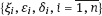are independent.

Vector β should be estimated based on observations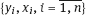.

In both logistic model with errors in the covariates (1.2), (1.3), and (1.5) and binary GLM with errors in the covariates (1.2), (1.4), and (1.5), exact assumptions about basic values are the following:

Random variables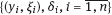are independent.

Vector β has to be estimated based on observations.

From a general point of view, the model with measurement errors consists of three parts:

(a)the regression model that links the response variable with unobservable ξ and observable z regressors, respectively;

(b)the measurement error model that connects ξ with the observable surrogate variable x;

(c)the distribution model of ξ .

In particular, the binary model (1.2), (1.4), and (1.5) gives: (1.2) and (1.4) to be the regression model in which there is no regressor z observable without errors; and (1.5) to be the model of measurement errors. In regards to the distribution of regressors ξi,one can assume, e.g., that the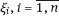are independent and identically distributed with normal distribution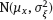, wherein the parameters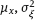(also called nuisance parameters) can be either known or unknown.

A regressor z can be included in the risk model as follows:

Here,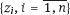are independent random vectors in Rq, and γRq is a vector of additional regression parameters. The estimators of the regression parameters β and γ are constructed based on observations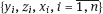in frames of the model (1.2), (1.5), and (1.6). Within the radio-epidemiological risk models, the vector zi components may present the age of person i as well as his/her gender along with individual features of the person. Usually the regressor z is the categorical variable, namely the one that takes discrete values.

## 1.1Structural and functional models, linear, and nonlinear models

In this section, we assume for simplicity that the observed part of regressors z to be absent in underlying measurement error models.

Consider the regression model linking the response yi with the regressors ξi. This model is called structural if the true values of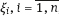, are random, moreover, they are independent and identically distributed in Rd. Usually, we know the form of the regressor ξ distribution, i.e., the probability density function (pdf) of ξ is known up to certain parameters. Those values (nuisance parameters) can be either known or unknown. If they are known, the distribution of ξ is given exactly.

For example, the structural logistic model (1.2), (1.4), and (1.5) usually requires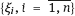to be independent and identically distributed random variables with common lognormal distribution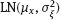, where the nuisance parameters μξR and σξ > 0 are unknown.

Unlike the structural model, the functional regression model assumes the true values ofto be nonrandom. Within the functional errors-in-variables models, the true regressor values ξi become the nuisance parameters; their number grows as the sample size n increases making it difficult to perform research.

Actually, the structural models impose stringent restrictions on the behavior of regressor values. Thus, the assumption that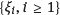is a sequence of independent identically distributed random variables with finite variance ensures, using the law of large numbers, the existence of finite limits for expressions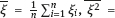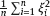and even for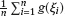, where g is a Borel measurable function such that |g(t)| ≤ const(1 + t2), t R.

In the framework of the functional models, if, e.g., it is required only to have additional stabilization of the expressions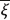and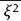, then it does not follow the existence of finite limit for the average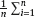sin ξi.

The choice between the structural and functional models relies on an accurate analysis of the measurement procedure. In epidemiology, the structural models are more popular, while modeling physical or chemical experiment where monotonously changing regressor ξ takes place is more relevant to the functional models.

We give an example of the functional model. In the adiabatic expansion, the gas pressure and gas volume are related to each other according to Boyle’s law:

Denote

Then the response y is related linearly to the regressor ξ:

Suppose that we observe y and ξ with additive errors

Naturally, regressors ξi = ln Vi can be supposed nonrandom because the adiabatic expansion affects on gas volume Vi being increased in time. Thus, the observation model (1.8) is naturally assumed to be the functional one, since the values ξi are nonrandom although unobservable. The errors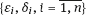are assumed independent. By observations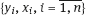the regression parameters β0 and β1 are estimated and next the parameters of equation (1.7), namely c = eβ0 and γ = −β1, are estimated as well.

Note that in terms of the original variables pi and Vi, we have a model with multiplicative errors

Here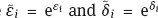are the multiplicative errors;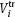is the true value of gas volume;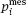and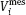are measured values of the pressure and volume, respectively. As this, the unknown valuesare assumed to be nonrandom.

Now, we consider an example of the structural model. Let us investigate the crime rate η in dependence on the average annual income ξ in a certain district of a country. This dependence we model using a given regression function η = f(ξ, β), where β is the vector of regression paramers. We randomly select the area i (e.g., the area of a big city), measure the crime rate (e.g., the number of registered crimes per capita), and take into account the average annual income ξi (e.g., by interviewing residents). For obvious reasons, the measurement will be inaccurate. One can assume that measurement errors are additive:

The regression model (1.1) in which the function f depends linearly on the regressor ξi is called linear. All other models of the regression of response y on the regressor ξ are called nonlinear. These are, in particular, the model (1.1) in which the regression function f depends nonlinearly (e.g., polynomially) on ξi, and the binary model (1.2) with any dependence of the odds function λ on ξi. The linear measurement error models will be studied in Chapter 2, and the nonlinear ones in all subsequent chapters.

## 1.2Classical measurement error and Berkson error

As already noted, the error δi is called the classical one in the context of the model (1.5), if ξi and δi are stochastically independent. The error describes instrumental measurements when some physical quantity to be measured using a device is characterized by a certain fluctuation error.

There is also the Berkson measurement model

Here, ξi is the true value of regressor (random and unobservable), xi is the result of the observation (random),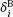is the Berkson error (centered), and it is known that xi andare stochastically independent. The model was named after the American Joseph Berkson (1899–1982), who first examined it in 1950.

It seems that one can transform the model (1.14) to the classical model (1.5) as follows:

The Berkson model occurs particularly in situations where the observation xi is formed by averaging. Imagine that some part of the observations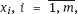2 ≤ m < n is an average quantity xc:

Then

Since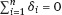, and due to symmetry

Hence the correlation coefficients are

The values xc and δi are uncorrelated. And δi are almost uncorrelated when m is large, that is,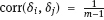tends to 0. Therefore, in the model (1.17), the values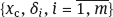can be considered approximately uncorrelated. If the probability of ξi are normal, then these variables are close to be independent.

This reasoning shows that the model (1.17), in a certain approximation, can be considered as the Berkson model (1.14). The latter can be realized when the entire sample x1,…, xn is divided into several groups. Inside each group, the data have been taken as a result of appropriate averaging, namely when one assigns the observation xi to an average which is close to the arithmetic mean of the corresponding values of the true regressor. We will often deal with such situations in the measurement of exposure doses within radiation risk models.

The Berkson and classical measurement errors can be compared with regard to the efficient estimation of regression parameters. Consider the structural model in two modifications: the first will have the classical errors (1.5) and the second will just have the Berkson ones (1.14), and let

For the first and second modifications, we construct the adequate estimates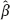cl andB of the regression parameter β (we will explain later how to construct such estimates). Then, the deviation ofcl from the true value will (very likely) be more than the corresponding deviation ofB

Here we use the Euclidean norm. The inequality (1.24) is qualitative in its nature and indicates the following trend: for the same level of errors, the classical errors stringently complicate the efficient estimation compared with the Berkson ones. Our experience with the binary model (1.2) and (1.4) confirms this conclusion.

## 1.3Explicit and implicit models

The models discussed above are explicit regression models in which the response (dependent variable) and the regressor (independent variable) are separated variables. The implicit models are more general where all variables under observation are treated equally. These models have the form

Here the latent variables ηi belong to Rm m ≥ 2, γi are the random (classical) measurement errors, the regression parameter β belongs to a parameter set ΘRp, the link function G : Rm × ΘR is known. One has to estimate the vector β by the observations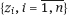.

Thus, the true values ηi lie on a given hypersurface

In fact, we want to retrieve this surface using the observed points. We impose the restriction m ≥ 2 in order to have at least two scalar variables, components of the vector η, among which there are some regression relations.

The implicit model (1.25) might be either the functional one where the ηi are nonrandom points on the surface Sβ, or the structural one where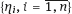are independent and identically distributed on the surface Sβ.

Here is an example of an implicit model. Suppose that we have to restore a circle using the observations of points on it. For the true points ηi = (xi, yi)T, it holds that

and the curve Sβ = {(x; y)TR2: (xx0)2 + (yy0)2 = r2} is just the circle.

It should be noted that the explicit model (1.1) and (1.5) can be transformed to the implicit one. For this purpose, denote αi = f(ξi, β), G(αi, ξi; β) = αif(ξi, β),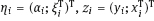, and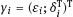. Relation (1.25) describing the implicit model holds true for the new variables. After such transformation, the response and the regressors are treated on an equal basis.

## 1.4Estimation methods

In regression errors-in-variables models, there are several reasonable estimation methods. Some of them are consistent, i.e., they yield estimatorsn of the parameter β that converge in probability to the true value β as the sample size tends to infinity. Others yield estimators with significant deviation from β; there are also estimators with reduced deviation. For small and moderate samples, the inconsistent estimators may even be advantageous because the consistent ones sometimes converge to β too slowly. The data of cohort radioepidemiologic studies include samples of rather moderate size, because if the number n of surveyed persons can reach tens of thousands then the number of oncological cases, fortunately, will be sufficiently smaller (about a hundred). From this point of view, the most promising methods are those that significantly reduce the deviationnβ of the estimators compared with “rough” estimation methods.

### 1.4.1Naive estimators

The naive estimation method constructs the estimators by algorithms that lead to consistent estimation in case of the absence of measurement errors, i.e., when the regressor is observed precisely.

Start with either the nonlinear regression model (1.1) and (1.5) or the model (1.1) and (1.14) with Berkson error. We can construct the naive estimator by ordinary least squares method using the objective function

The corresponding naive estimator is as follows:

and define the estimatorOLS as one of the solutions to the equation

The estimatorsOLS andOLS do not differ very much from each other. The naive researcher would follow this way if he/she knows nothing about the theory of measurement errors in covariates. The mentioned researcher just neglects the existence of such errors and constructs the estimator to be consistent when the errors are absent.

Now, consider the binary model (1.2) and (1.5), where the odds function λ has the form either (1.3) or (1.4). Should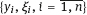be observed the likelihood function will be equal to

with λi = λ(ξi, β). The loglikelihood function is

May 26, 2018 | Posted by in NUCLEAR MEDICINE | Comments Off on Measurement error models
Premium Wordpress Themes by UFO Themes